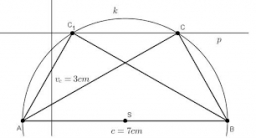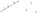# Diagonal intersect

isosceles trapezoid ABCD with length bases | AB | = 6 cm, CD | = 4 cm is divided into 4 triangles by the diagonals intersecting at point S. How much of the area of the trapezoid are ABS and CDS triangles?

Correct result:

r1 =  0.36
r2 =  0.16

#### Solution:We would be pleased if you find an error in the word problem, spelling mistakes, or inaccuracies and send it to us. Thank you!Tips to related online calculators
Check out our ratio calculator.

#### You need to know the following knowledge to solve this word math problem:

We encourage you to watch this tutorial video on this math problem:

## Next similar math problems:

• Find the 10Find the value of t if 2tx+5y-6=0 and 5x-4y+8=0 are perpendicular, parallel, what angle does each of the lines make with the x-axis, find the angle between the lines?
• Three points 2The three points A(3, 8), B(6, 2) and C(10, 2). The point D is such that the line DA is perpendicular to AB and DC is parallel to AB. Calculate the coordinates of D.
• Perpendicular projectionDetermine the distance of a point B[1, -3] from the perpendicular projection of a point A[3, -2] on a straight line 2 x + y + 1 = 0.
• Ascend vs. descentWhich function is growing? a) y = 2-x b) y = 20 c) y = (x + 2). (-5) d) y = x-2
• Vector v4Find the vector v4 perpendicular to vectors v1 = (1, 1, 1, -1), v2 = (1, 1, -1, 1) and v3 = (0, 0, 1, 1)
• Calculate 6Calculate the distance of a point A[0, 2] from a line passing through points B[9, 5] and C[1, -1].
• DodecagonCalculate the size of the smaller of the angles determined by lines A1 A4 and A2 A10 in the regular dodecagon A1A2A3. .. A12. Express the result in degrees.
• Decide 2Decide whether points A[-2, -5], B[4, 3] and C[16, -1] lie on the same line
• Vector perpendicularFind the vector a = (2, y, z) so that a⊥ b and a ⊥ c where b = (-1, 4, 2) and c = (3, -3, -1)
• Vector equationLet’s v = (1, 2, 1), u = (0, -1, 3) and w = (1, 0, 7) . Solve the vector equation c1 v + c2 u + c3 w = 0 for variables c1 c2, c3 and decide weather v, u and w are linear dependent or independent
• Angle of the body diagonalsUsing vector dot product calculate the angle of the body diagonals of the cube.
• Coordinates of a centroindLet’s A = [3, 2, 0], B = [1, -2, 4] and C = [1, 1, 1] be 3 points in space. Calculate the coordinates of the centroid of △ABC (the intersection of the medians).
• Parametric formCalculate the distance of point A [2,1] from the line p: X = -1 + 3 t Y = 5-4 t Line p has a parametric form of the line equation. ..
• Set of coordinatesConsider the following ordered pairs that represent a relation. {(–4, –7), (0, 6), (5, –3), (5, 2)} What can be concluded of the domain and range for this relation?
• Find the 5Find the equation of the circle with center at (1,20), which touches the line 8x+5y-19=0
• Two peopleTwo straight lines cross at right angles. Two people start simultaneously at the point of intersection. John walking at the rate of 4 kph in one road, Jenelyn walking at the rate of 8 kph on the other road. How long will it take for them to be 20√5 km apa
• Points collinearShow that the point A(-1,3), B(3,2), C(11,0) are col-linear.